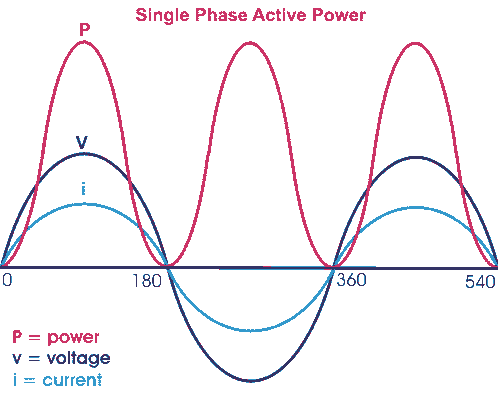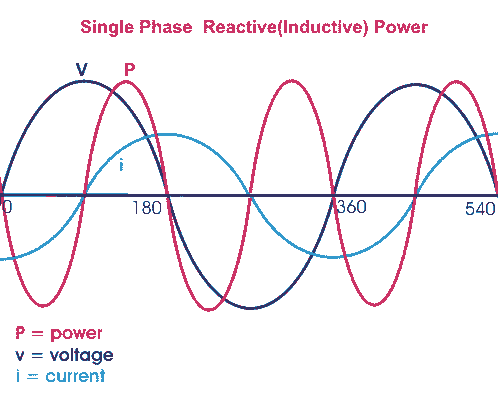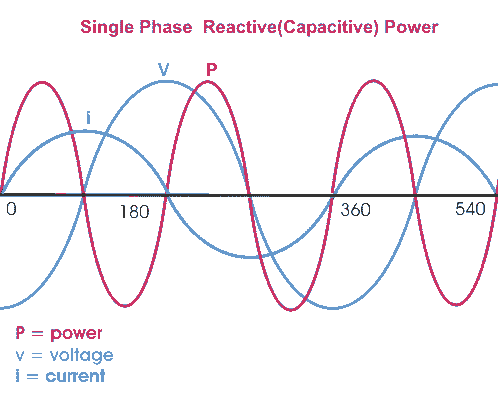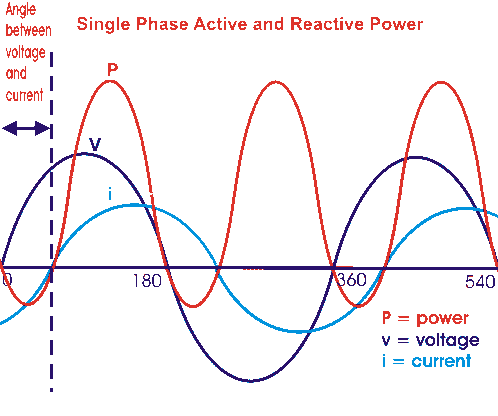Home » » Single Phase Power

# Single Phase Power

A single phase transmission system is practically not available but still we should know first the basic concept of single phase power before going through modern 3 phase power system.

Before going to details about single phase power, let's try to understand different Parameters of Electrical Power System

Three basic parameters of Electrical Power System are resistance, inductance and capacitance.

#### Resistance

The resistance of power circuit or simply resistor, consumes ohmic energy. While electric current flows through a resistor there will not be any phase difference between the voltage and current, that means electric current and voltage are in same phase the phase angle between them is zero. If I current flows through a resistance R for t seconds then total energy consumed by the resistor is I2.R.t. This power is known as active power

#### Inductance

Inductance of the system or simply inductor stores magnetic field energy during positive half cycle and gives away during negative half cycle of single phase power supply. If a current 'I' flows through a coil of inductance L Henri, the energy stored in the coil in form of magnetic field is given by

 1 L.I2 2

The power associated with an inductance is reactive power.

#### Capacitance

Capacitance of the system or simply capacitor, stores electric field energy during positive half cycle and give away during negative half cycle of supply. The energy stored between two parallel metallic plates of potential difference V and capacitance across them C, is expressed as

 1 C.V2 2

This energy is stored in form of electric field. The power associated with an capacitance is also reactive power.

## Active Power and Reactive Power

Let us consider an single phase power circuit in which current lags behind the voltage by an angle φ.
Let the instantaneous voltage v = Vm.sinωt
then the instantaneous current can be expressed as i = Im. sin(ωt - φ).
Where,Vm and Im are the maximum values of sinusoidal system voltage and current respectively
The instantaneous power is given by

p = v.i = Vm.sinωt. Im. sin(ωt - φ)

 = 1 Vm. Im.2.sinωt. sin(ωt - φ) 2
 = 1 Vm. Im.[{cosωt. cos(ωt - φ) + sinωt. sin(ωt - φ)} – { cosωt. cos(ωt - φ) - sinωt. sin(ωt - φ)}] 2
 = 1 Vm. Im.[cos{ωt - (ωt - φ)} - cos{ωt + (ωt - φ)}] 2
 = 1 Vm. Im.[cos φ - cos(2ωt - φ)] 2

## Active Power

### Resistive Power

Let's take the condition first where the single phase power circuit is fully resistive, that means the phase angle between voltage and current φ = 0; and hence

 p = 1 Vm. Im.[1 - cos2ωt] 2From the above equation it is clear that whatever may be the value of ωt the value of cos2ωt can not be greater than 1 hence the value of p can not be negative. The value of p is always positive irrespective of the instantaneous direction of voltage v and current i, that means the energy is flowing in its conventional direction e.i. from source to load and p is the rate of energy consumption by the load and this is called active power. As this power is consumed due to resistive effect of an electrical circuit hence sometimes it is also calledresisteive power

## Reactive Power

### Inductive Power

Now consider a situation when the single phase power circuit is fully inductive, that means the current lags behind the voltage by an angle φ = + 90o. Putting φ = + 90o

 in the equation p = 1 Vm. Im.[cos φ - cos(2ωt - φ)] we get, 2
 p = 1 Vm. Im.[0 - cos(2ωt - 90o)] 2
 = − 1 Vm. Im.sin2ωt 2In the above expression, it is found that the power is flowing in alternative directions. From 0o to 90o it will have negative half cycle, from 90o to 180o it will have positive half cycle, from 180oto 270o it will have again negative half cycle and from 270o to 360o it will have again positive half cycle. Therefore this power is alternating in nature with a frequency, double of supply frequency. As the power is flowing in alternating direction e.i. from source to load in one half cycle and from load to source in next half cycle the average value of this power is zero. Therefore this power does not do any useful work. This power is known as reactive power. As the above explained reactive power expression is related to fully inductive circuit, this power is also called inductive power

This can be concluded as if the circuit is purely inductive, energy will be stores as magnetic field energy during positive half cycle and give away during negative half cycle and rate at which this energy changes, expressed as reactive power of inductor or simply inductive power and this power will have equal positive and negative cycle and the net value will be zero.

### Capacitive Power

Let us now consider the single phase power circuit is fully capacitive, that is the current leads the voltage by 90o, therefore φ = - 90o.

 Putting this value in the equation p = 1 Vm. Im.[cos φ - cos(2ωt - φ)] we get, 2
 p = 1 Vm. Im.[0 - cos{2ωt - ( - 90o)}] 2
 = 1 Vm. Im.sin2ωt 2Hence in the expression ofcapacitive power, it is also found that the power is flowing in alternative directions. From 0o to 90o it will have positive half cycle, from 90o to 180o it will have negative half cycle, from 180o to 270o it will have again positive half cycle and from 270o to 360o it will have again negative half cycle. So this power is also alternating in nature with a frequency, double of supply frequency. Therefore, as inductive power, the capacitive power does not do any useful work. This power is also a reactive power.

### Active Power and Reactive Power

The power equation can be re-written as

p = Vm. Im.[cosφ – cos(2ωt - φ)]

= Vm. Im.[cosφ – cosφ cos2ωt - sinφ sin2ωt]

= Vm. Im.cosφ(1 – cos2ωt) – Vm. Imsinφsin2ωt

This above expression has two consonants; first one is Vm. Im.cosφ(1 – cos2ωt) which never goes negative as because value of (1 – cos2ωt) always greater or equal zero but can not have a negative value.This portion of the single phase power equation represents the expression of reactive power which is alternatively known as real power or true power. The average of this power will obviously have some non zero value means the power physically does some useful work and that is why this power is also called real power or sometimes it is referred as true power. This portion of the power equation represents the reactive power which is alternatively known as real power or true power.
Second term is Vm. Im.sinφsin2ωt which will have negative and positive cycles. Hence average of this component is zero. This component is known as reactive component as it travels back and forth on the line without doing any useful work.

Both active power and reactive power have same dimensions of watts but to emphasize the fact that reactive component represents a non-active power, it is measured in terms of volt-amperes reactive or in short VAR.

--